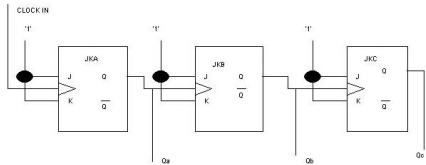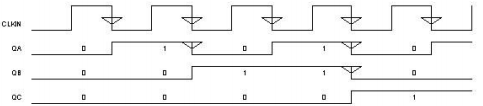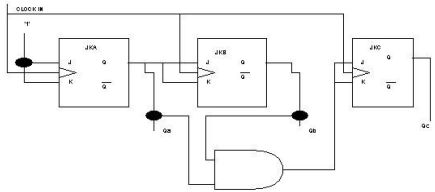## Asynchronous and synchronous logic design, Electrical Engineering

Assignment Help:

One of the simplest circuits is the asynchronous or ' ripple' counter. Below is shown the circuit diagram of a simple 3 stage ripple counter.The operation of this circuit is based on the fact that the truth table for the JK flip flop is only valid if the clock waveform is falling, i.e. 1->0. Assume the outputs are all zero, the flip flops will not change until the clock on each flip flop falls. The clock in waveform has just fallen  ,since the JKa inputs are logic '1' the device will toggle and the output will invert i.e. Qa=1. Flip flop B will not change because the clock waveform on B has risen    (0->1) and these devices only functions on a falling edge. The clock in waveform has fallen again, so Qa toggles again (i.e. Qa =0), this has just produced a falling clock on JKb and Qb toggles (i.e. Qab=1) .The device has just counted from 000-> 001->010.The circuit is called a ripple counter because the clock pulse is slowly rippling through the JK's, hence asynchronous (Not at the same time!) .The limitations of the asynchronous counter is the speed of operation. A rough formula for the maximum speed is when the clock changes before the output changes i.e.

F =  1 / n x propagation delay

where n = number of stages, propagation delay of one JK

A better technique is to use a synchronous design where all the JK are clocked together so the maximum frequency is only limited by the propagation delay of 1 JK.The circuit appears to be complex in design, however it is easily realised by using state diagrams. The maximum frequency of operation is again roughly calculated by considering the frequency at which the output just changes before the clock in changes.

F = 1/ Propagation delay

#### Applied mechanics, State whether Lamis theorem is applicable for more than ...

State whether Lamis theorem is applicable for more than three coplanar concurrent forces?

#### Rftfrfr, #questionfrfrfrfr..

#questionfrfrfrfr..

#### Phase lead controller design and digital implementation, The arm of a hydra...

The arm of a hydraulic robot is controlled as shown in the block diagram below: The arm dynamics are represented by: Dynamic specification for the arm requires:

#### Dc chopper - power supplies , DC chopper This is  one stage  conversio...

DC chopper This is  one stage  conversion  device  which is used for direct conversion of fixed  de voltage at a level to an adjustable dc voltage  at another  level.

#### Explain interrupts of 8085, Explain Interrupts of 8085. a) Maskable  in...

Explain Interrupts of 8085. a) Maskable  interrupt b)  Vectored interrupt c)  Non maskable interrupt d) Hardware interrupt e)  Software interrupt

Q. Advantage of digital technology? The primary advantage of digital technology is the low cost, simplicity, and versatility of the digital building blocks. Because digital sig

#### Microcontroller interfacing, The conveyor system is driven by a 240 Volt 50...

The conveyor system is driven by a 240 Volt 50 Hz AC motor requiring a continuous 1 Amp supply. The conveyor has single direction operation. The loading mechanism for the winder

#### Circuit theorems, limitations and applications of following circuit theorem...

limitations and applications of following circuit theorems: telleans theorem superposition theorem norton theorem compensation theorem millman theorem reciprocity theorem maximum p

mosfet

#### Find the voltage, Q. The current sources in Figure are given to be I A = 3...

Q. The current sources in Figure are given to be I A = 30 A and I B = 50 A. For the values of R 1 = 20 ,R2 = 40 , and R3 = 80 , find: (a) The voltage V. (b) The current